Latest Banking jobs   »

# Quantitative Aptitude Quiz For SBI PO/Clerk Mains 2022- 29th December

Direction (1-5): In the given questions, two quantities are given, one as ‘Quantity 1’ and another as ‘Quantity 2’. You have to determine relationship between two quantities and choose the appropriate option:
(a) Quantity 1 > Quantity 2
(b) Quantity 1 ≥ Quantity 2
(c) Quantity 2 > Quantity 1
(d) Quantity 2 ≥ Quantity 1
(e) Quantity 1 = Quantity 2 or Relation cannot be established

Q1. Quantity 1: Find the speed of train (in m/s) if the train takes 20 sec to completely cross another train coming from opposite direction of twice the length & speed both as that of first train. The first train can cross a platform 100 m long in 30 sec.
Quantity 2: Find the speed of train (in m/s) if the train overtakes another train running at a speed of 20 m/s in 70 sec while the latter train can cross a platform of same length in 25 sec. Length of first train is 60% less than that of latter train. (latter train is faster)

Q2. Quantity 1: Find the number of blue balls in the bag if the probability of getting a red ball when 3 balls are drawn at random from the bag having 15 balls is 45/91. If there would be no blue balls, this probability would be 5/11. The bag contains red, blue & green color balls and the blue balls are minimum in the bag.
Quantity 2: Find the number of blue balls in the bag if the bag contains ‘x’ red balls & ‘y’ blue balls such that x²-3x-10=0 and probability of drawing 2 red balls from the bag at random is 2/9.

Q3. Quantity 1: Find the value of (a+b-c)² if(a+b)²=25=b²+c²; a < b < c & a, b, c ∈ N
Quantity 2: Find the value of (a+b-c)² if a :b=1:1; a is 40% less than c and average of b & c is 4.

Q4. Quantity 1: In what time (min) the tank will be filled completely if all the pipes are opened simultaneously. There are 3 pipes of which 2 are inlet and other is outlet. If there is only 1 inlet (which is the most efficient), then tank will be filled by both the pipes in 30 min. The inlet pipes alone take 10 & 12 min respectively to fill the tank. All pipes are opened till tank is filled.
Quantity 2: In what time (min) the tank will be filled completely if all the pipes are opened simultaneously. Pipe A, B & C alone can fill the tank in 15, 12 & 10 min respectively. Pipes are opened in a pair for a minute & no pipe works for more than 2 min continuously. (consider minimum time taken)

Q5. Quantity 1: Curved surface area of sphere is 616 sq.m. Find curved surface area of cylinder (sq.m.) if the sphere is melted to form a cylinder of height 28/3 m.
Quantity 2: Curved surface area of sphere is 616 sq.m. Find curved surface area of cylinder (sq.m.) if the cylinder can completely fit inside the sphere such that top & bottom of cylinder lies on surface of sphere. Radius of cylinder = 7 m.

Directions (6-10): In the given questions, two quantities are given, one as ‘Quantity I’ and another as ‘Quantity II’. You have to determine relationship between two quantities and choose the appropriate option:

Q6. 5X men can complete a work in X/2 days while 2Y men can complete same work in 4Y/5 days.
Quantity I: Value of ‘Y+20’.
Quantity II: Value of ‘1.25X’
(a) Quantity I ≥ Quantity II
(b) Quantity I = Quantity II or No relation
(c) Quantity I > Quantity II
(d) Quantity I < Quantity II
(e) Quantity I ≤ Quantity II

Q7. Rs. X is invested in a scheme which offers 15% p.a. at C.I. Interest earned after two years is Rs.3870.
Quantity I: ‘Value of ‘A’. Rs. 2X is invested in a scheme which offers A% p.a. at C.I. and interest earned after two years is Rs. 10,560
Quantity II: Value of ‘B’. Rs. (X+6,000) is invested in a scheme which offers B% p.a. at C.I. and amounts to Rs. 28,125
(a) Quantity I > Quantity II
(b) Quantity I = Quantity II or No relation
(c) Quantity I ≥ Quantity II
(d) Quantity I < Quantity II
(e) Quantity I ≤ Quantity II

Q8. Total surface area of a cylinder is 200% more than that of its sum of area of base and top of cylinder. Volume of cylinder is 2156 cm³
Quantity I: Volume of cone whose base radius and height is same as that of radius and height of cylinder respectively.
Quantity II: Volume of hemisphere whose radius is same as that of radius of cylinder.
(a) Quantity I > Quantity II
(b) Quantity I = Quantity II or No relation
(c) Quantity I ≥ Quantity II
(d) Quantity I < Quantity II
(e) Quantity I ≤ Quantity II

Q9. There are ‘A’ numbers of cubes each having side 2 cm is melted and re-casted into a cylinder of radius 7 cm and height 8 cm.
Quantity I: Value of ‘A+77’.
Quantity II: Value of ‘1.5A’
(a) Quantity I ≥ Quantity II
(b) Quantity I = Quantity II
(c) Quantity I ≤ Quantity II
(d) Quantity I < Quantity II
(e) Quantity I > Quantity II

Q10. ‘B’ kg of Rs. 36 per/kg rice that mixed with 8 kg of Rs. 42 per/kg rice, in order to earn profit of 10% while selling the mixture at Rs. 44 per/kg.
Quantity I: Value of ‘B+20’.
Quantity II: Value of ‘6B’
(a) Quantity I < Quantity II
(b) Quantity I ≤ Quantity II
(c) Quantity I > Quantity II
(d) Quantity I ≥ Quantity II
(e) Quantity I = Quantity II

Directions (11-15): The following questions are accompanied by two statements A and B. You have to determine which statements(s) is/are sufficient/necessary to answer the questions.
(a) Statement A alone is sufficient to answer the question, but statement B alone is not sufficient to answer the questions.
(b) Statement B alone is sufficient to answer the question, but statement A alone is not sufficient to answer the question.
(c) Both the statements taken together are necessary to answer the questions, but neither of the statements alone is sufficient to answer the question.
(d) Either statement A or statement B by itself is sufficient to answer the question.
(e) Statements A and B taken together are not sufficient to answer the question.

Q11. Calculate SP of article A, If shopkeeper earns a profit of 20% on its CP.
(A) He marked up the price Rs. 75 more than its CP.
(B) Had he give discount of 10% on its MP, he would earn Rs. 7.5 less rupees than actual.

Q12. Find the speed of boat
(A) time taken to cover a distance of 18 km, first in upstream, then in downstream is 4 ½ hours.
(B) If the speed of current gets doubled, then time taken to cover 18 km, first in downstream, then in upstream is 7 ⅕ hours.

Q13. Find the volume of right angle cone ?
(A) Curved surface area of cone 18π units and T.S.A. of cone 50% more than curved surface area.
(B) Volume of cylinder of twice the radius as that of cone & √3 time the height as that cone is 324π cubic units.

Q14. Rana told that, his son after x years will be ⅕th of his age, Rana’s wife Negi told that their only son after x years will be ¼th of her age. What is sum of age of Rana and his wife after x years.
(A) The age difference between husband and wife is 9 years.
(B) Value of x is 3.

Q15. A group of people have to collect Rs. 800 for a party and each one of them have to contribute equally. How many peoples were in the group.
(A) Each one of them paid a multiple of 10 and number of people in group was also multiple of 10 and total number of people were less than money paid by each of them.
(B) If 4 people left the group, then each one have to pay an additional Rs. 10

Solutions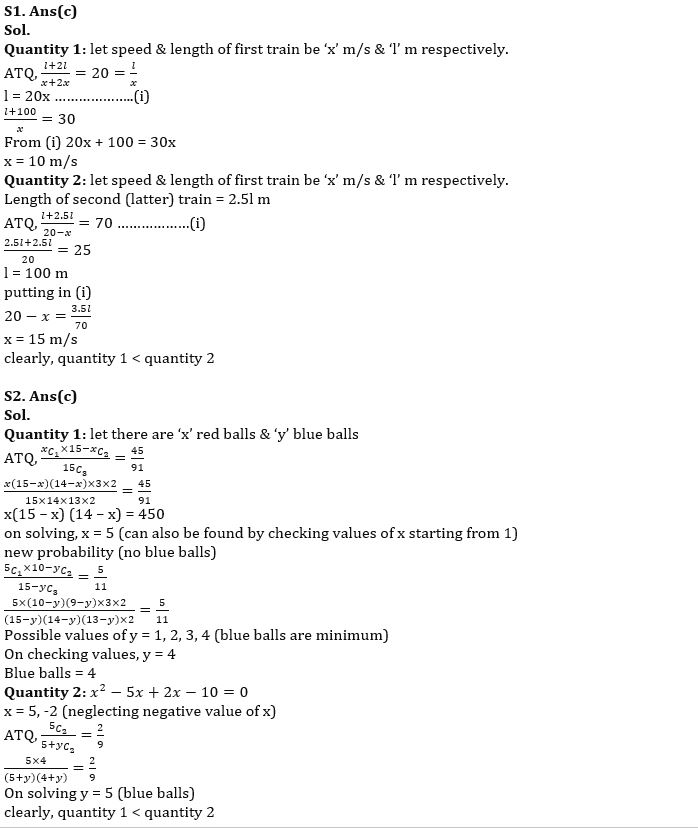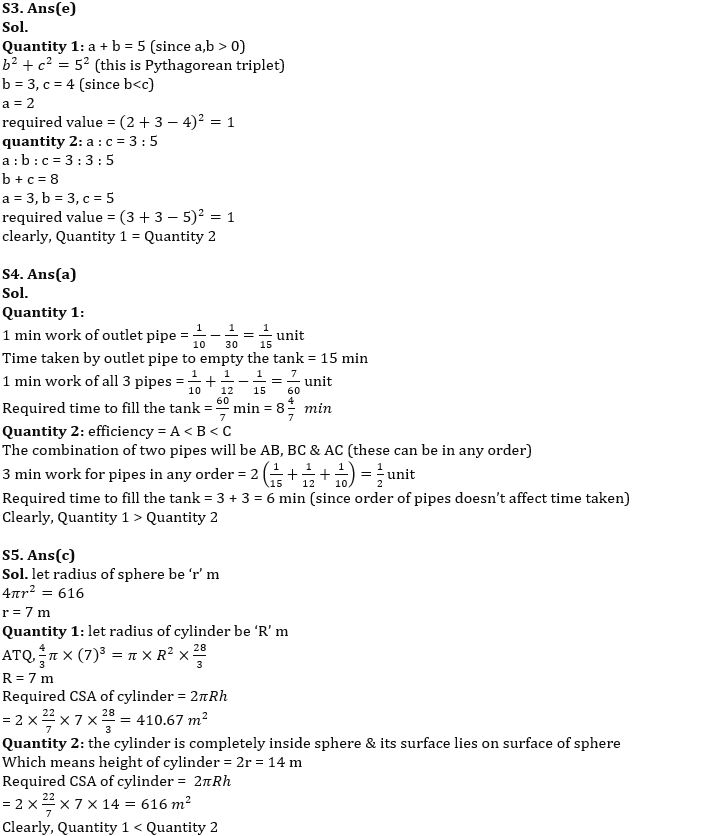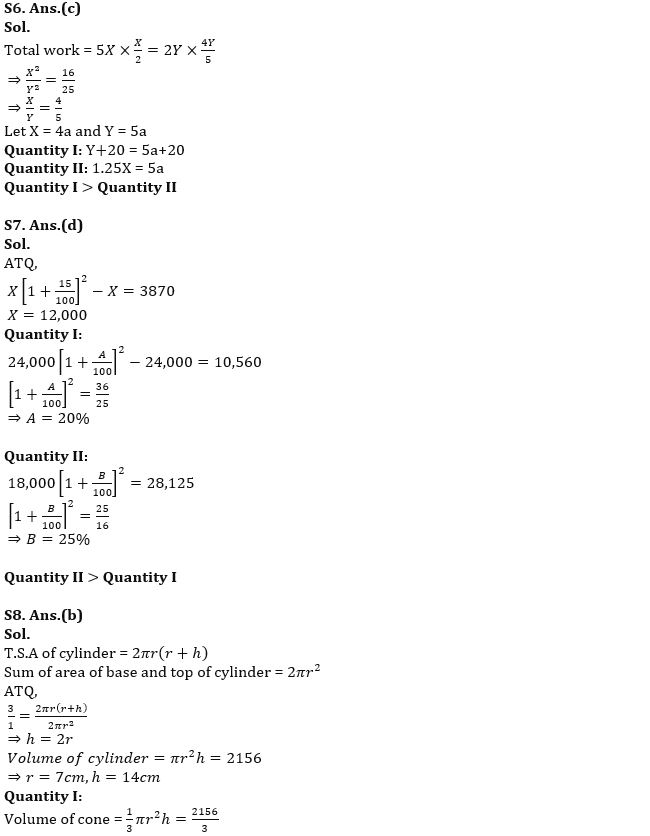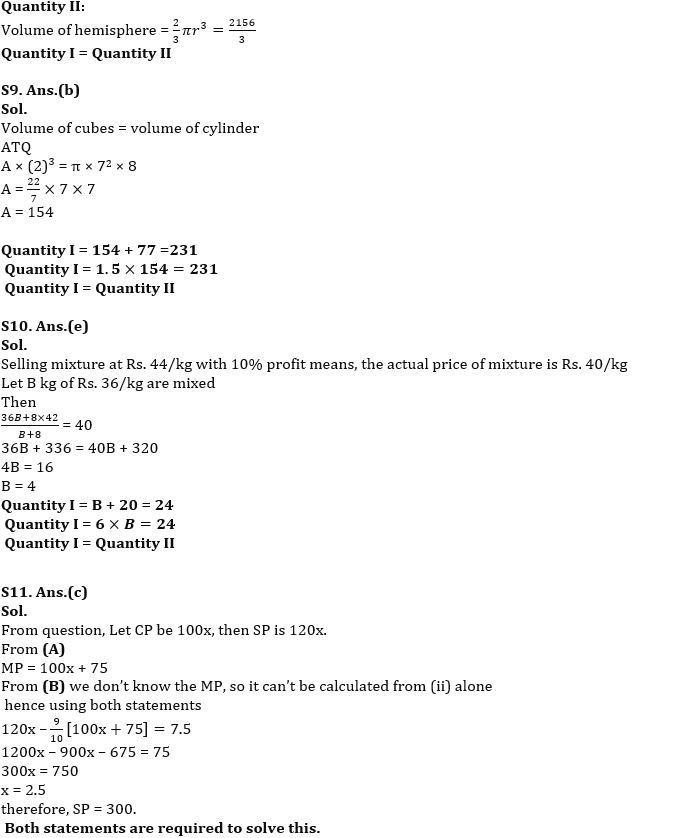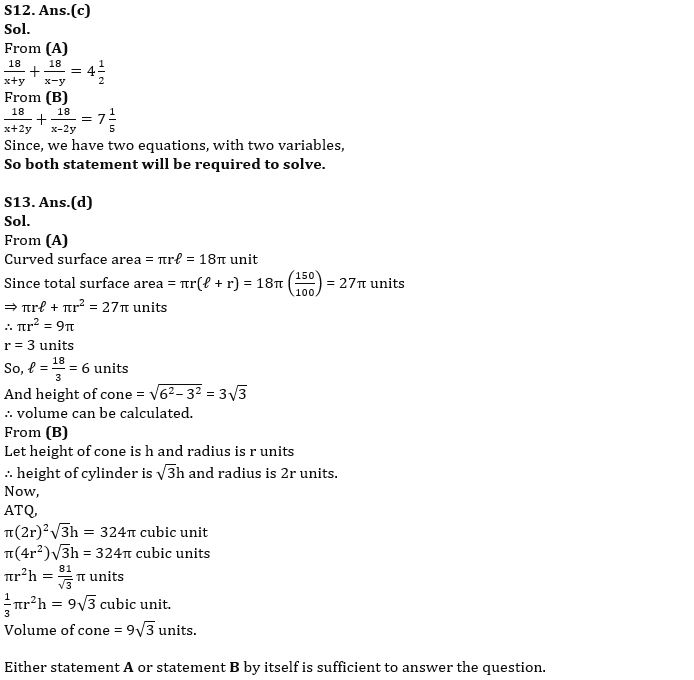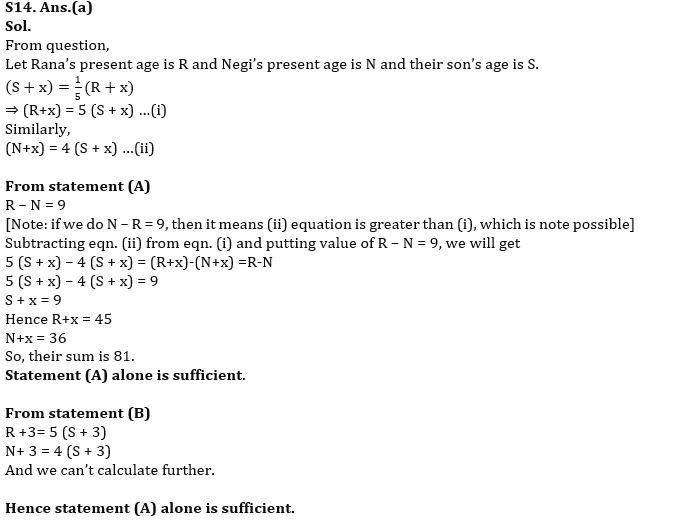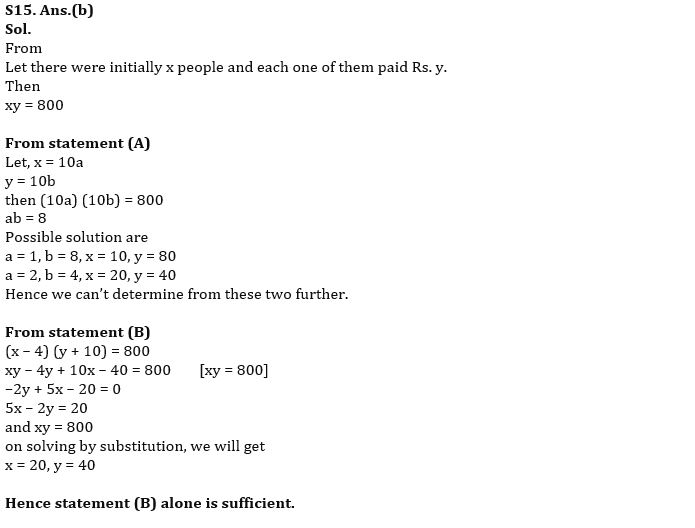#### Congratulations!Union Budget 2023-24: Free PDF